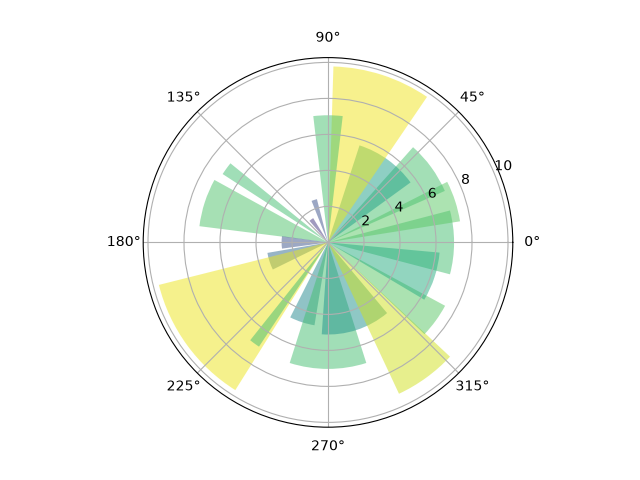# Bar chart on polar axis#

Demo of bar plot on a polar axis.

import matplotlib.pyplot as plt
import numpy as np

# Fixing random state for reproducibility
np.random.seed(19680801)

# Compute pie slices
N = 20
theta = np.linspace(0.0, 2 * np.pi, N, endpoint=False)
width = np.pi / 4 * np.random.rand(N)